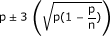1. * Callus growth was measured by the difference between the final and initial weight of embryogenic cultures after a 1 month proliferation cycle. The initial weight was calibrated at 120 ± 10 mg. Each datum corresponds to the mean ± SD of 4 measurements. We performed an ANOVA followed by the Tukey HSD test to identify significant differences between the means of all treatments. Values with different letters are significantly different at P ≤ 0.05. ** Transformation efficiency was analyzed by the proportion (p) of transformed calli (p = x/n), where x is the number of transformed calli and n the number of co-cultivated calli. A 3 δ confidence limit for binomial distribution was calculated using the formulawith a level of confidence of 99%. Each value is the mean of 4 replicates.